# Parameter Selection for HDBSCAN*¶

While the HDBSCAN class has a large number of parameters that can be set on initialization, in practice there are a very small number of parameters that have significant practical effect on clustering. We will consider those major parameters, and consider how one may go about choosing them effectively.

## Selecting `min_cluster_size`¶

The primary parameter to effect the resulting clustering is `min_cluster_size`. Ideally this is a relatively intuitive parameter to select – set it to the smallest size grouping that you wish to consider a cluster. It can have slightly non-obvious effects however. Let’s consider the digits dataset from sklearn. We can project the data into two dimensions to visualize it via t-SNE.

```digits = datasets.load_digits()
data = digits.data
projection = TSNE().fit_transform(data)
plt.scatter(*projection.T, **plot_kwds)
```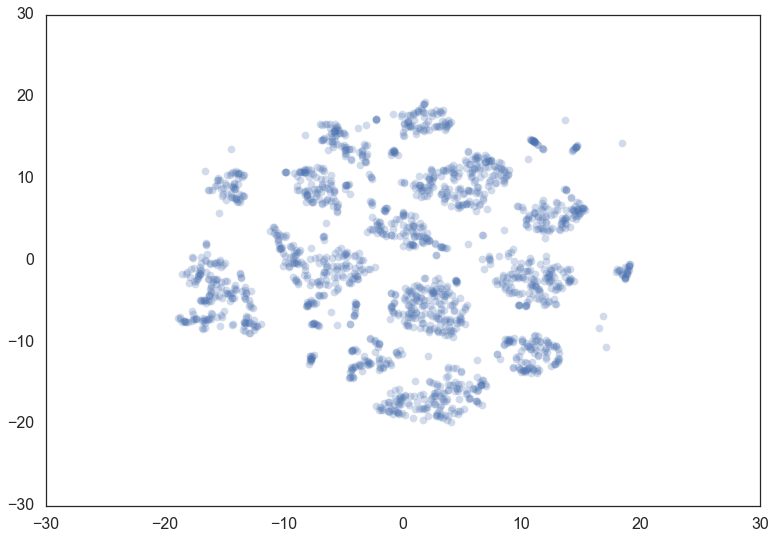If we cluster this data in the full 64 dimensional space with HDBSCAN* we can see some effects from varying the `min_cluster_size`.

We start with a `min_cluster_size` of 15.

```clusterer = hdbscan.HDBSCAN(min_cluster_size=15).fit(data)
color_palette = sns.color_palette('Paired', 12)
cluster_colors = [color_palette[x] if x >= 0
else (0.5, 0.5, 0.5)
for x in clusterer.labels_]
cluster_member_colors = [sns.desaturate(x, p) for x, p in
zip(cluster_colors, clusterer.probabilities_)]
plt.scatter(*projection.T, s=50, linewidth=0, c=cluster_member_colors, alpha=0.25)
```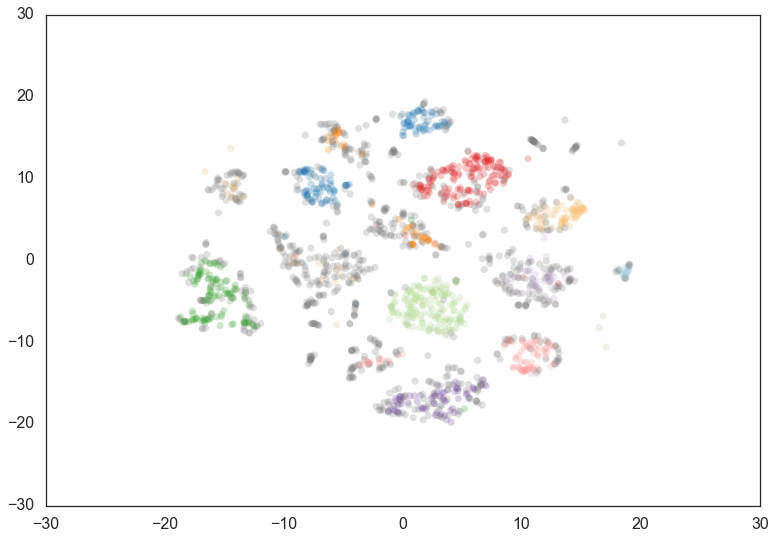Increasing the `min_cluster_size` to 30 reduces the number of clusters, merging some together. This is a result of HDBSCAN* reoptimizing which flat clustering provides greater stability under a slightly different notion of what constitutes a cluster.

```clusterer = hdbscan.HDBSCAN(min_cluster_size=30).fit(data)
color_palette = sns.color_palette('Paired', 12)
cluster_colors = [color_palette[x] if x >= 0
else (0.5, 0.5, 0.5)
for x in clusterer.labels_]
cluster_member_colors = [sns.desaturate(x, p) for x, p in
zip(cluster_colors, clusterer.probabilities_)]
plt.scatter(*projection.T, s=50, linewidth=0, c=cluster_member_colors, alpha=0.25)
```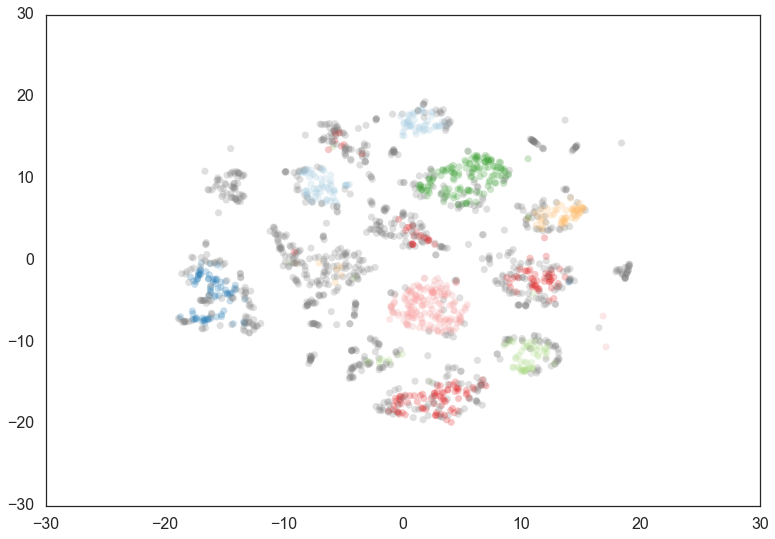Doubling the `min_cluster_size` again to 60 gives us just two clusters – the really core clusters. This is somewhat as expected, but surely some of the other clusters that we had previously had more than 60 members? Why are they being considered noise? The answer is that HDBSCAN* has a second parameter `min_samples`. The implementation defaults this value (if it is unspecified) to whatever `min_cluster_size` is set to. We can recover some of our original clusters by explicitly providing `min_samples` at the original value of 15.

```clusterer = hdbscan.HDBSCAN(min_cluster_size=60).fit(data)
color_palette = sns.color_palette('Paired', 12)
cluster_colors = [color_palette[x] if x >= 0
else (0.5, 0.5, 0.5)
for x in clusterer.labels_]
cluster_member_colors = [sns.desaturate(x, p) for x, p in
zip(cluster_colors, clusterer.probabilities_)]
plt.scatter(*projection.T, s=50, linewidth=0, c=cluster_member_colors, alpha=0.25)
```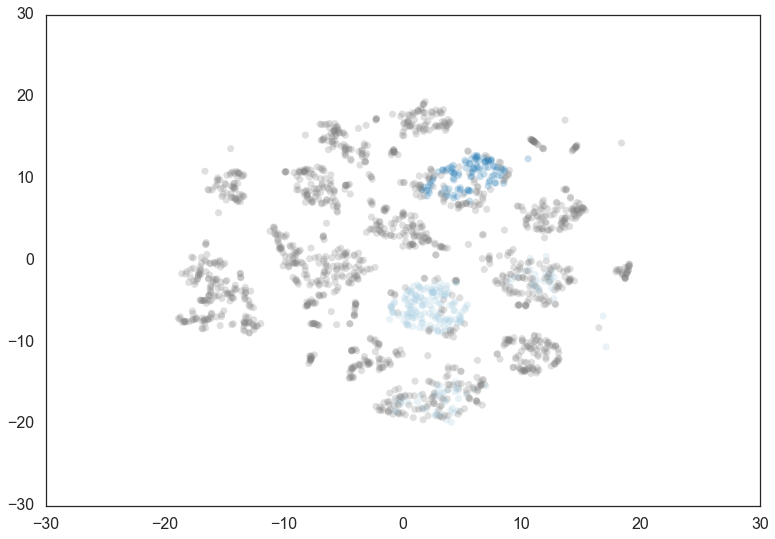```clusterer = hdbscan.HDBSCAN(min_cluster_size=60, min_samples=15).fit(data)
color_palette = sns.color_palette('Paired', 12)
cluster_colors = [color_palette[x] if x >= 0
else (0.5, 0.5, 0.5)
for x in clusterer.labels_]
cluster_member_colors = [sns.desaturate(x, p) for x, p in
zip(cluster_colors, clusterer.probabilities_)]
plt.scatter(*projection.T, s=50, linewidth=0, c=cluster_member_colors, alpha=0.25)
```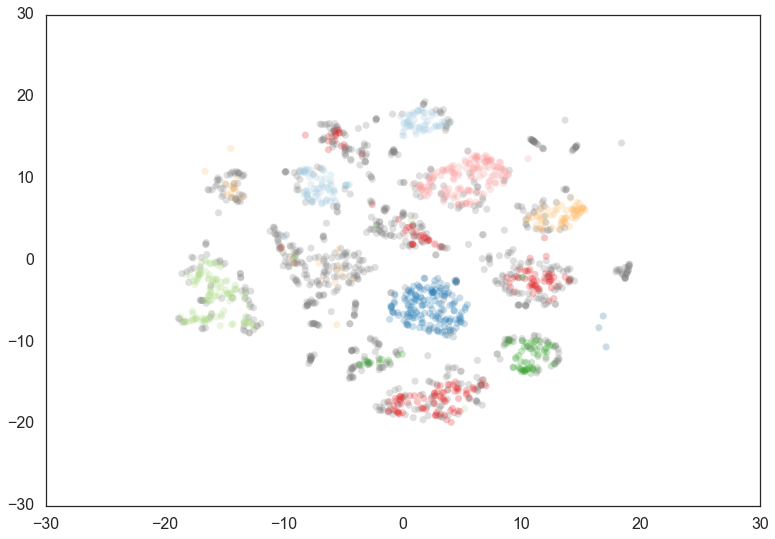As you can see this results in us recovering something much closer to our original clustering, only now with some of the smaller clusters pruned out. Thus `min_cluster_size` does behave more closely to our intuitions, but only if we fix `min_samples`.

If you wish to explore different `min_cluster_size` settings with a fixed `min_samples` value, especially for larger dataset sizes, you can cache the hard computation, and recompute only the relatively cheap flat cluster extraction using the `memory` parameter, which makes use of joblib

## Selecting `min_samples`¶

Since we have seen that `min_samples` clearly has a dramatic effect on clustering, the question becomes: how do we select this parameter? The simplest intuition for what `min_samples` does is provide a measure of how conservative you want you clustering to be. The larger the value of `min_samples` you provide, the more conservative the clustering – more points will be declared as noise, and clusters will be restricted to progressively more dense areas. We can see this in practice by leaving the `min_cluster_size` at 60, but reducing `min_samples` to 1.

Note: adjusting `min_samples` will result in recomputing the hard comptuation of the single linkage tree.
```clusterer = hdbscan.HDBSCAN(min_cluster_size=60, min_samples=1).fit(data)
color_palette = sns.color_palette('Paired', 12)
cluster_colors = [color_palette[x] if x >= 0
else (0.5, 0.5, 0.5)
for x in clusterer.labels_]
cluster_member_colors = [sns.desaturate(x, p) for x, p in
zip(cluster_colors, clusterer.probabilities_)]
plt.scatter(*projection.T, s=50, linewidth=0, c=cluster_member_colors, alpha=0.25)
```
```<matplotlib.collections.PathCollection at 0x120978438>
```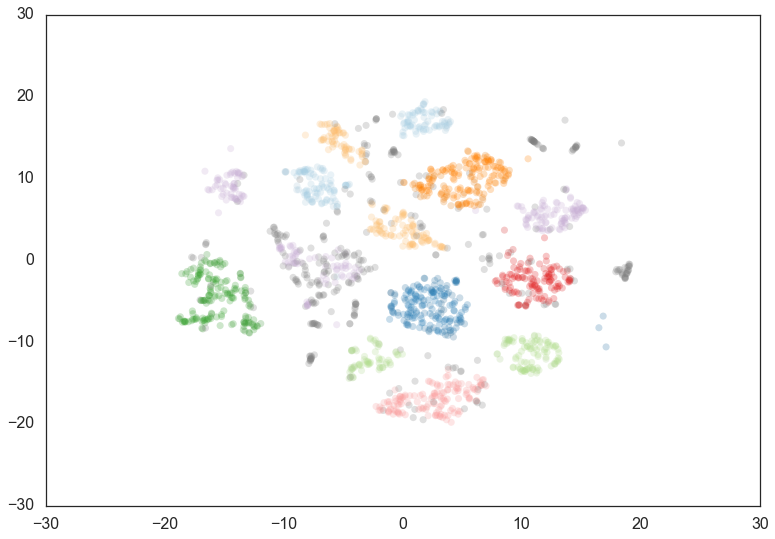Now most points are clustered, and there are much fewer noise points. Steadily increasing `min_samples` will, as we saw in the examples above, make the clustering progressively more conservative, culminating in the example above where `min_samples` was set to 60 and we had only two clusters with most points declared as noise.

## Selecting `alpha`¶

A further parameter that effects the resulting clustering is `alpha`. In practice it is best not to mess with this parameter – ultimately it is part of the `RobustSingleLinkage` code, but flows naturally into HDBSCAN*. If, for some reason, `min_samples` is not providing you what you need, stop, rethink things, and try again with `min_samples`. If you still need to play with another parameter (and you shouldn’t), then you can try setting `alpha`. The `alpha` parameter provides a slightly different approach to determining how conservative the clustering is. By default `alpha` is set to 1.0. Increasing `alpha` will make the clustering more conservative, but on a much tighter scale, as we can see by setting `alpha` to 1.3.

Note: adjusting `alpha` will result in recomputing the hard comptuation of the single linkage tree.
```clusterer = hdbscan.HDBSCAN(min_cluster_size=60, min_samples=15, alpha=1.3).fit(data)
color_palette = sns.color_palette('Paired', 12)
cluster_colors = [color_palette[x] if x >= 0
else (0.5, 0.5, 0.5)
for x in clusterer.labels_]
cluster_member_colors = [sns.desaturate(x, p) for x, p in
zip(cluster_colors, clusterer.probabilities_)]
plt.scatter(*projection.T, s=50, linewidth=0, c=cluster_member_colors, alpha=0.25)
```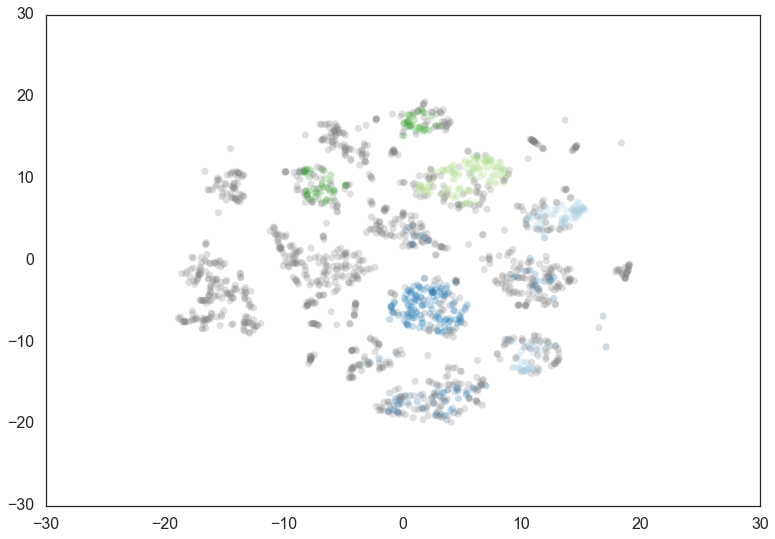## Leaf clustering¶

HDBSCAN supports an extra parameter `cluster_selection_method` to determine how it selects flat clusters from the cluster tree hierarchy. The default method is `'eom'` for Excess of Mass, the algorithm described in How HDBSCAN Works. This is not always the most desireable approach to cluster selection. If you are more interested in having small homogeneous clusters then you may find Excess of Mass has a tendency to pick one or two large clusters and then a number of small extra clusters. In this situation you may be tempted to recluster just the data in the single large cluster. Instead, a better option is to select `'leaf'` as a cluster selection method. This will select leaf nodes from the tree, producing many small homogeneous clusters. Note that you can still get variable density clusters via this method, and it is also still possible to get large clusters, but there will be a tendency to produce a more fine grained clustering than Excess of Mass can provide.

## Allowing a single cluster¶

In contrast, if you are getting lots of small clusters, but believe there should be some larger scale structure (or the possibility of no structure), consider the `allow_single_cluster` option. By default HDBSCAN* does not allow a single cluster to be returned – this is due to how the Excess of Mass algorithm works, and a bias towards the root cluster that may occur. You can override this behaviour and see what clustering would look like if you allow a single cluster to be returned. This can alleviate issue caused by there only being a single large cluster, or by data that is essentially just noise. For example, the image below shows the effects of setting `allow_single_cluster=True` in the bottom row, compared to the top row which used default settings.